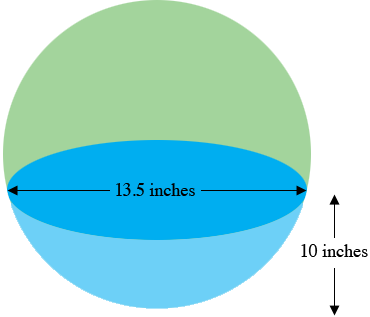SEARCH HOMEMath Central Quandaries & QueriesQuestion from Danny: How many gallons of water in a glass bowl with the dimensions of 10 inches high and 13.5 inches wide?Hi Danny,

By a "glass bowl" I assume the shape of the bowl is spherical as in the diagram below.The section shaded blue is called a spherical cap and if you know some calculus you can find its volume as a volume of revolution. The volume is given by

$V = \frac{\pi \; h^2}{3}\left( 3r - h\right)$

where in your case $h = 10$ inches and $r = \large \frac{13.5}{2}$ inches. I got $V = 1073.38$ cubic inches. (you should check my arithmetic.)

To find the volume in gallons you could type

what is 1073.38 cubic inches in gallons

into the Google search window or, I'm a Mac user so I said

Hey Siri, what's 1073.38 cubic inches in gallons.

PennyMath Central is supported by the University of Regina and The Pacific Institute for the Mathematical Sciences.